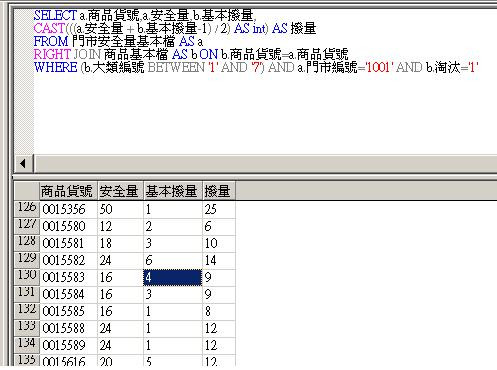#1

## MS SQL將數商品數量除以2，但必須符合基本撥量的倍數

Dear 各位先進

``````SELECT a.商品貨號,a.安全量,b.基本撥量
FROM 門市安全量基本檔 AS a
RIGHT JOIN 商品基本檔 AS b ON b.商品貨號=a.商品貨號
WHERE (b.大類編號 BETWEEN '1' AND '7') AND a.門市編號='1001' AND b.淘汰='1'
``````

(圖1)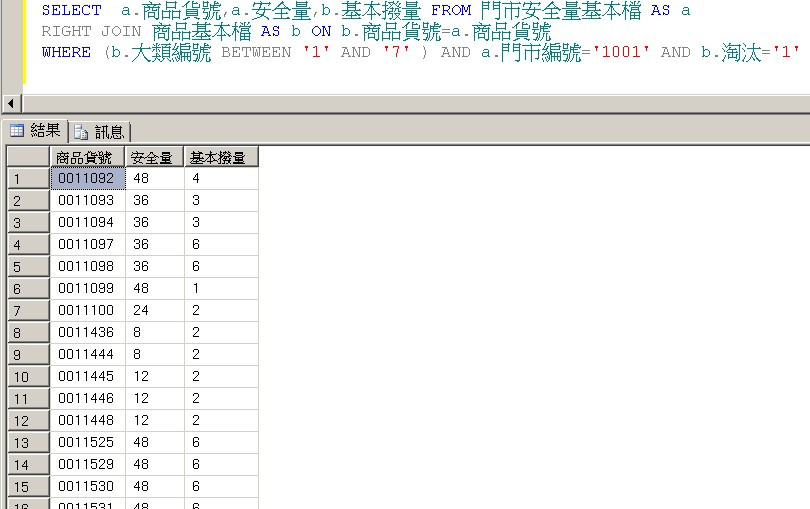(圖2)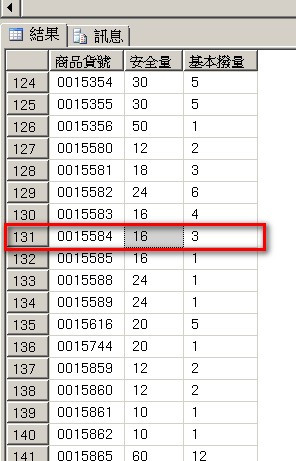sjzgo iT邦新手 4 級 ‧ 2018-06-22 10:34:16 檢舉

### 2 個回答

4
``````with CTE as (
SELECT a.商品貨號,a.安全量,b.基本撥量
FROM 門市安全量基本檔 AS a
RIGHT JOIN 商品基本檔 AS b ON b.商品貨號=a.商品貨號
WHERE (b.大類編號 BETWEEN '1' AND '7') AND a.門市編號='1001' AND b.淘汰='1'
)
select [商品貨號],
[安全量] [原安全量],
[基本撥量],
case when ([安全量]/2) % [基本撥量] = 0 then [安全量]/2
else [基本撥量] * (([安全量]/2)/[基本撥量]+1)
end  [新安全量]
from CTE
``````

11092 48 4 24
1103 36 3 18
15584 16 3 9

# 線上測試連結

sjzgo iT邦新手 4 級 ‧ 2018-06-22 12:18:29 檢舉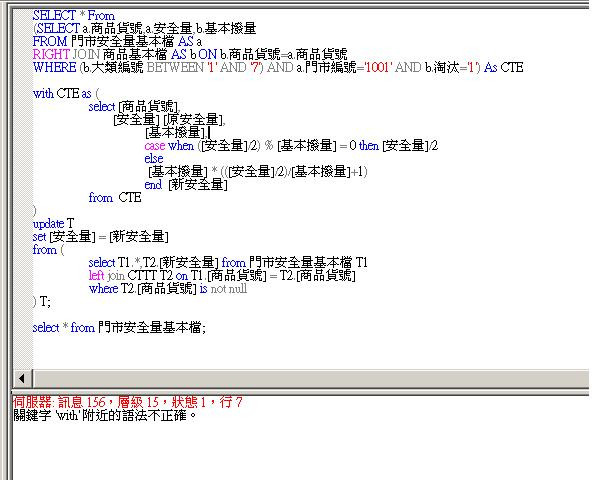``````SELECT * From
(SELECT a.商品貨號,a.安全量,b.基本撥量
FROM 門市安全量基本檔 AS a
RIGHT JOIN 商品基本檔 AS b ON b.商品貨號=a.商品貨號
WHERE (b.大類編號 BETWEEN '1' AND '7') AND a.門市編號='1001' AND b.淘汰='1') As CTE

with CTE as (
select [商品貨號],
[安全量] [原安全量],
[基本撥量],
case when ([安全量]/2) % [基本撥量] = 0 then [安全量]/2
else
[基本撥量] * (([安全量]/2)/[基本撥量]+1)
end  [新安全量]
from  CTE
)
update T
set [安全量] = [新安全量]
from (
select T1.*,T2.[新安全量] from 門市安全量基本檔 T1
left join CTTT T2 on T1.[商品貨號] = T2.[商品貨號]
where T2.[商品貨號] is not null
) T;

select * from 門市安全量基本檔;
``````

``````update T
set [安全量] = [新安全量]
from (
SELECT a.商品貨號,a.安全量,b.基本撥量 ,
case when (a.安全量/2) % b.基本撥量 = 0 then a.安全量/2
else b.基本撥量 * ((a.安全量/2)/b.基本撥量+1)
end  [新安全量]
FROM 門市安全量基本檔 AS a
RIGHT JOIN 商品基本檔 AS b ON b.商品貨號=a.商品貨號
WHERE (b.大類編號 BETWEEN '1' AND '7') AND a.門市編號='1001' AND b.淘汰='1'
) T
``````

``````select * into 門市安全量基本檔_0622_back from 門市安全量基本檔
``````
sjzgo iT邦新手 4 級 ‧ 2018-06-22 14:20:07 檢舉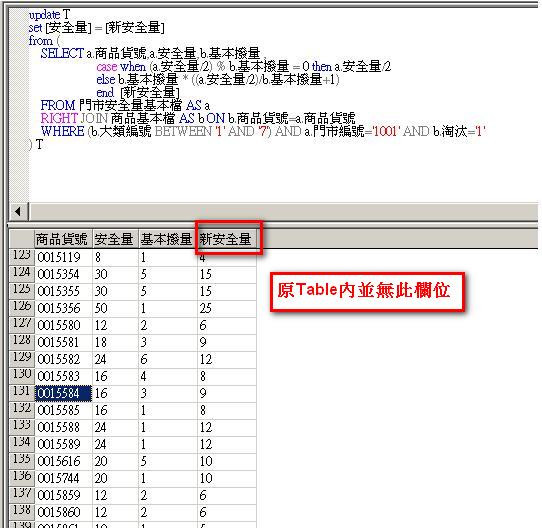sjzgo iT邦新手 4 級 ‧ 2018-06-22 17:33:12 檢舉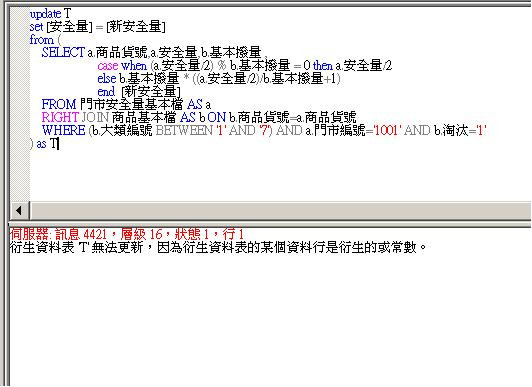``````select * into #temp_table from (
SELECT a.商品貨號 取代商品貨號,a.安全量,b.基本撥量 ,
case when (a.安全量/2) % b.基本撥量 = 0 then a.安全量/2
else b.基本撥量 * ((a.安全量/2)/b.基本撥量+1)
end  [新安全量]
FROM 門市安全量基本檔 AS a
RIGHT JOIN 商品基本檔 AS b ON b.商品貨號=a.商品貨號
WHERE (b.大類編號 BETWEEN '1' AND '7') AND a.門市編號='1001' AND b.淘汰='1'
) T

update 門市安全量基本檔
set 安全量 = (select [新安全量] from #temp_table  where 商品貨號 = 取代商品貨號)
where 商品貨號 in (select 取代商品貨號 from #temp_table )

``````

Google 了一下，發現 暐翰 大大的範例可以改成這樣，不過不知道在 SQL SERVER 2000 上可不可以正確執行。

``````update a
set a.[安全量] =
case when (a.安全量/2) % b.基本撥量 = 0 then a.安全量/2
else b.基本撥量 * ((a.安全量/2)/b.基本撥量+1)
end
FROM 門市安全量基本檔 AS a
RIGHT JOIN 商品基本檔 AS b ON b.商品貨號=a.商品貨號
WHERE (b.大類編號 BETWEEN '1' AND '7') AND a.門市編號='1001' AND b.淘汰='1'
``````
sjzgo iT邦新手 4 級 ‧ 2018-06-22 23:16:24 檢舉

sjzgo iT邦新手 4 級 ‧ 2018-06-25 17:34:21 檢舉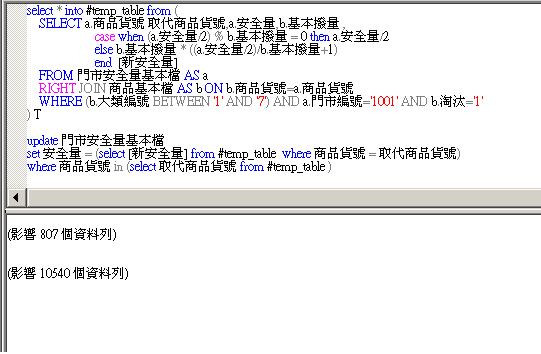sjzgo iT邦新手 4 級 ‧ 2018-06-25 17:46:24 檢舉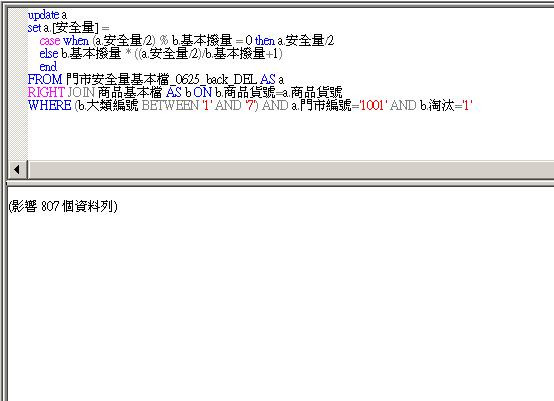sjzgo iT邦新手 4 級 ‧ 2018-06-25 18:12:32 檢舉

sjzgo iT邦新手 4 級 ‧ 2018-06-26 10:40:50 檢舉

fysh711426

0

（安全量 + (基本撥量 - 1）)/ 2

(48+(2-1))/2 = 24

(16+(3-1))/2 = 9

SELECT a.商品貨號,a.安全量,b.基本撥量,

CAST(((a.安全量 + b.基本撥量-1) / 2) AS int) AS 撥量

FROM 門市安全量基本檔 AS a
RIGHT JOIN 商品基本檔 AS b ON b.商品貨號=a.商品貨號
WHERE (b.大類編號 BETWEEN '1' AND '7') AND a.門市編號='1001' AND b.淘汰='1'

sjzgo iT邦新手 4 級 ‧ 2018-06-22 18:00:08 檢舉

sjzgo iT邦新手 4 級 ‧ 2018-06-22 18:07:25 檢舉